# relative-equilibrium.pptx

28 May 2023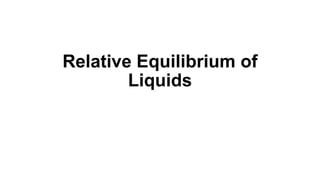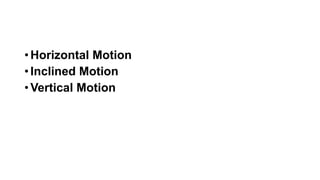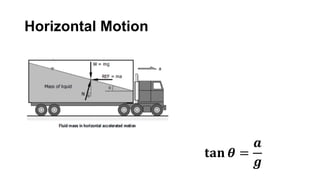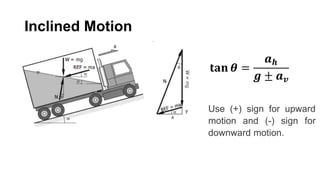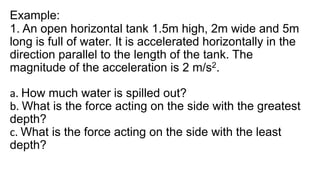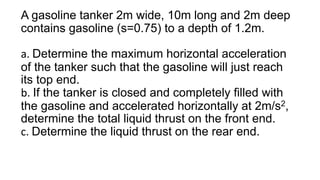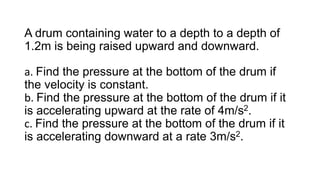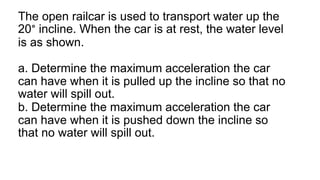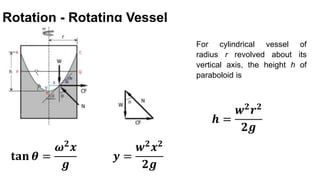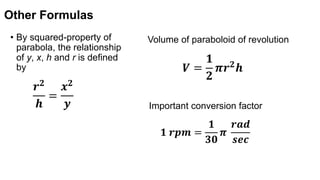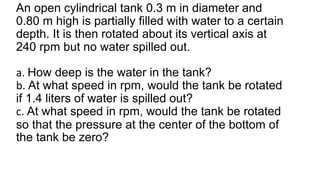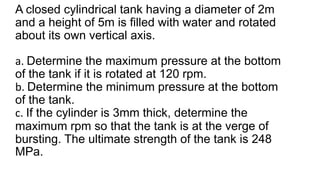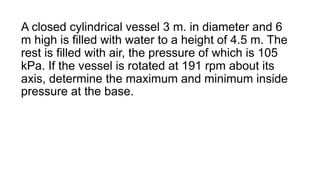1 sur 14

### relative-equilibrium.pptx

• 2. • Horizontal Motion • Inclined Motion • Vertical Motion
• 4. Inclined Motion 𝐭𝐚𝐧 𝜽 = 𝒂𝒉 𝒈 ± 𝒂𝒗 Use (+) sign for upward motion and (-) sign for downward motion.
• 5. Vertical Motion 𝒑 = 𝜸𝒉(𝟏 ± 𝒂 𝒈 ) Use (+) for upward motion Use (-) for downward motion Also note that a is positive for acceleration and negative for deceleration.
• 6. Example: 1. An open horizontal tank 1.5m high, 2m wide and 5m long is full of water. It is accelerated horizontally in the direction parallel to the length of the tank. The magnitude of the acceleration is 2 m/s2. a. How much water is spilled out? b. What is the force acting on the side with the greatest depth? c. What is the force acting on the side with the least depth?
• 7. A gasoline tanker 2m wide, 10m long and 2m deep contains gasoline (s=0.75) to a depth of 1.2m. a. Determine the maximum horizontal acceleration of the tanker such that the gasoline will just reach its top end. b. If the tanker is closed and completely filled with the gasoline and accelerated horizontally at 2m/s2, determine the total liquid thrust on the front end. c. Determine the liquid thrust on the rear end.
• 8. A drum containing water to a depth to a depth of 1.2m is being raised upward and downward. a. Find the pressure at the bottom of the drum if the velocity is constant. b. Find the pressure at the bottom of the drum if it is accelerating upward at the rate of 4m/s2. c. Find the pressure at the bottom of the drum if it is accelerating downward at a rate 3m/s2.
• 9. The open railcar is used to transport water up the 20° incline. When the car is at rest, the water level is as shown. a. Determine the maximum acceleration the car can have when it is pulled up the incline so that no water will spill out. b. Determine the maximum acceleration the car can have when it is pushed down the incline so that no water will spill out.
• 10. Rotation - Rotating Vessel 𝐭𝐚𝐧 𝜽 = 𝝎𝟐 𝒙 𝒈 𝒚 = 𝒘𝟐 𝒙𝟐 𝟐𝒈 For cylindrical vessel of radius r revolved about its vertical axis, the height h of paraboloid is 𝒉 = 𝒘𝟐 𝒓𝟐 𝟐𝒈
• 11. Other Formulas • By squared-property of parabola, the relationship of y, x, h and r is defined by 𝒓𝟐 𝒉 = 𝒙𝟐 𝒚 Volume of paraboloid of revolution 𝑽 = 𝟏 𝟐 𝝅𝒓𝟐 𝒉 Important conversion factor 𝟏 𝒓𝒑𝒎 = 𝟏 𝟑𝟎 𝝅 𝒓𝒂𝒅 𝒔𝒆𝒄
• 12. An open cylindrical tank 0.3 m in diameter and 0.80 m high is partially filled with water to a certain depth. It is then rotated about its vertical axis at 240 rpm but no water spilled out. a. How deep is the water in the tank? b. At what speed in rpm, would the tank be rotated if 1.4 liters of water is spilled out? c. At what speed in rpm, would the tank be rotated so that the pressure at the center of the bottom of the tank be zero?
• 13. A closed cylindrical tank having a diameter of 2m and a height of 5m is filled with water and rotated about its own vertical axis. a. Determine the maximum pressure at the bottom of the tank if it is rotated at 120 rpm. b. Determine the minimum pressure at the bottom of the tank. c. If the cylinder is 3mm thick, determine the maximum rpm so that the tank is at the verge of bursting. The ultimate strength of the tank is 248 MPa.
• 14. A closed cylindrical vessel 3 m. in diameter and 6 m high is filled with water to a height of 4.5 m. The rest is filled with air, the pressure of which is 105 kPa. If the vessel is rotated at 191 rpm about its axis, determine the maximum and minimum inside pressure at the base.